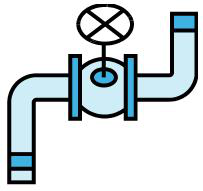* Log In to use the Calculate function * Become a Member!

###### Water Pipeline & Pump Design:• Calculate the velocity head loss for a pipe under pressure flow.

Minor Loss Equivalent Length
• Calculate the minor loss equivalent length in a pipe under pressure flow, (for use in calculating head losses from valves, fittings, etc.) in equivalent length of straight pipe.
• Calculate the friction head loss for a water distribution line or force main.  This calculation is based on the Hazen-Williams equation using length of pipe.  The total pipe length in this case can also include the equivalent length of valves and fittings to account for minor losses.
Pipe Discharge
• Calculate the discharge Q for a pipe given the head on the pipe.  This calculation is based on Hazen-Williams equation setting the head on the pipe equal to the friction head loss in which the length of pipe can include the equivalent length to account for minor losses from valves and fittings. The velocity head loss in this case is considered negligible.
• Calculate the total dynamic head (TDH) requirements of the water distribution line or sewer force main to determine the required pump size.
Pump Design Variables
• Calculate the horsepower requirements of the pump and motor from the total dynamic head requirements of the transmission line.
• Convert head (H), (absolute pressure) expressed in ft or m, to psi or kPa, or convert from psi or kPa to ft or m.  Convert English units to English units or metric units to metric units.
Gage Pressure
• Convert gage pressure to absolute pressure, or absolute pressure to gage pressure.  Convert English units to English units or metric units to metric units.
Thrust Blocks - Bends
• Calculate the required area for a concrete thrust block on a bend in a water distribution line.
Thrust Blocks - Branch
• Calculate the required area for a thrust block on a tee in a water distribution line.
Frictional Resist/Soil Pressure
• Calculate the frictional resistance or soil pressure variables for a pipeline.  Both variables are required to determine restrained joint length.
Restrained Joint - Length
• Calculate the restrained joint length requirements for a water distribution line or force main.
Design Problem 1
• Calculate the discharge from the open end of a pressurized water pipe accounting for minor losses.

 Start Using The Calculations >>> Become A Member Now!